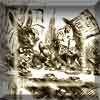#### You may also like### A Problem of Time

Consider a watch face which has identical hands and identical marks for the hours. It is opposite to a mirror. When is the time as read direct and in the mirror exactly the same between 6 and 7?### Eight Dominoes

Using the 8 dominoes make a square where each of the columns and rows adds up to 8### Fit for Photocopying

Explore the relationships between different paper sizes.

# Similar Perimeter

##### Age 14 to 16 Short Challenge Level:

The lengths of the sides of a triangle A are 3, 4, and 6.

Triangle B is similar to triangle A. One of the sides of triangle B has length 12.

What are the possible perimeters that triangle B could have?

This problem is taken from the World Mathematics Championships
You can find more short problems, arranged by curriculum topic, in our short problems collection.